# Electrochemistry

Electrochemistry is a branch of chemistry involving the study of interrelationships between electricity and chemical reactions. The chemical reactions generally take place in solution, at the interface between an electron conductor (a metal or semiconductor) and an ion conductor (the electrolyte), and involve electron transfer between the electrode and the electrolyte or species in solution.

If a chemical reaction is driven by an external applied voltage, as in electrolysis, or if a voltage is generated by a chemical reaction, as in a battery, the reaction is called an electrochemical reaction. Chemical reactions where electrons are transferred between molecules are called oxidation/reduction (redox) reactions.

Electrochemical reactions are valuable for many important applications. For example, they may be used to extract metals from their ores, or to coat objects with metals or metal oxides through electrodeposition. The redox reaction may be used to detect alcohol in drunken drivers or to measure glucose levels in the blood of diabetics. In nature, the generation of chemical energy through photosynthesis is an electrochemical process.

## History

### Developments from the sixteenth to eighteenth centuries

The sixteenth century marked the beginning of electrical understanding. During that century the English scientist William Gilbert spent 17 years experimenting with magnetism and, to a lesser extent, electricity. For his work on magnets, Gilbert became known as the "Father of Magnetism." He discovered various methods for producing and strengthening magnets.

In 1663, the German physicist Otto von Guericke created the first electric generator, which produced static electricity by applying friction in the machine. The generator was made of a large sulfur ball cast inside a glass globe, mounted on a shaft. The ball was rotated by means of a crank and a static electric spark was produced when a pad was rubbed against the ball as it rotated. The globe could be removed and used as source for experiments with electricity.

By the mid-eighteenth century, the French chemist Charles François de Cisternay du Fay discovered two types of static electricity, and that like charges repel each other whilst unlike charges attract. DuFay announced that electricity consisted of two fluids: "vitreous" (from the Latin for "glass"), or positive, electricity; and "resinous," or negative, electricity. This was the two-fluid theory of electricity, which was to be opposed by Benjamin Franklin's one-fluid theory later in the century.

Charles-Augustin de Coulomb developed the law of electrostatic attraction in 1781 as an outgrowth of his attempt to investigate the law of electrical repulsions as stated by Joseph Priestley in England.

In the late eighteenth century, the Italian physician and anatomist Luigi Galvani marked the birth of electrochemistry by establishing a bridge between chemical reactions and electricity on his essay "De Viribus Electricitatis in Motu Musculari Commentarius" (Latin for Commentary on the Effect of Electricity on Muscular Motion) in 1791 where he proposed a "nerveo-electrical substance" on biological life forms.

In his essay, Galvani concluded that animal tissue contained a here-to-fore neglected innate, vital force, which he termed "animal electricity," which activated nerves and muscles spanned by metal probes. He believed that this new force was a form of electricity in addition to the "natural" form produced by lightning or by the electric eel and torpedo ray as well as the "artificial" form produced by friction (i.e., static electricity).

Galvani's scientific colleagues generally accepted his views, but Alessandro Volta rejected the idea of an "animal electric fluid," replying that the frog's legs responded to differences in metal temper, composition, and bulk. Galvani refuted this by obtaining muscular action with two pieces of the same material.

### Nineteenth century

In 1800, William Nicholson and Johann Wilhelm Ritter succeeded in decomposing water into hydrogen and oxygen by electrolysis. Soon thereafter Ritter discovered the process of electroplating. He also observed that the amount of metal deposited and the amount of oxygen produced during an electrolytic process depended on the distance between the electrodes. By 1801 Ritter observed thermoelectric currents and anticipated the discovery of thermoelectricity by Thomas Johann Seebeck.

By the 1810s, William Hyde Wollaston made improvements to the galvanic pile. Sir Humphry Davy's work with electrolysis led to the conclusion that the production of electricity in simple electrolytic cells resulted from chemical action and that chemical combination occurred between substances of opposite charge. This work led directly to the isolation of sodium and potassium from their compounds and of the alkaline earth metals from theirs in 1808.

Hans Christian Ørsted's discovery of the magnetic effect of electrical currents in 1820 was immediately recognized as an epoch-making advance, although he left further work on electromagnetism to others. André-Marie Ampère quickly repeated Ørsted's experiment, and formulated them mathematically.

In 1821, Estonian-German physicist Thomas Johann Seebeck demonstrated the electrical potential in the juncture points of two dissimilar metals when there is a heat difference between the joints.

In 1827, the German scientist Georg Ohm expressed his law in this famous book Die galvanische Kette, mathematisch bearbeitet (The Galvanic Circuit Investigated Mathematically) in which he gave his complete theory of electricity.

In 1832, Michael Faraday's experiments led him to state his two laws of electrochemistry. In 1836 John Daniell invented a primary cell in which hydrogen was eliminated in the generation of the electricity. Daniell had solved the problem of polarization. In his laboratory he had learned that alloying the amalgamated zinc of Sturgeon with mercury would produce a better voltage.

William Grove produced the first fuel cell in 1839. In 1846, Wilhelm Weber developed the electrodynamometer. In 1866, Georges Leclanché patented a new cell which eventually became the forerunner to the world's first widely used battery, the zinc carbon cell.

Svante August Arrhenius published his thesis in 1884 on Recherches sur la conductibilité galvanique des électrolytes (Investigations on the galvanic conductivity of electrolytes). From his results the author concluded that electrolytes, when dissolved in water, become to varying degrees split or dissociated into electrically opposite positive and negative ions.

In 1886, Paul Héroult and Charles M. Hall developed a successful method to obtain aluminium by using the principles described by Michael Faraday.

In 1894, Friedrich Ostwald concluded important studies of the electrical conductivity and electrolytic dissociation of organic acids.

Walther Hermann Nernst developed the theory of the electromotive force of the voltaic cell in 1888. In 1889, he showed how the characteristics of the current produced could be used to calculate the free energy change in the chemical reaction producing the current. He constructed an equation, known as Nernst Equation, which related the voltage of a cell to its properties.

In 1898, Fritz Haber showed that definite reduction products can result from electrolytic processes if the potential at the cathode is kept constant. He also explained the reduction of nitrobenzene in stages at the cathode and this became the model for other similar reduction processes.

### The twentieth century

In 1902, The Electrochemical Society (ECS) was founded.

In 1909, Robert Andrews Millikan began a series of experiments to determine the electric charge carried by a single electron.

In 1923, Johannes Nicolaus Brønsted and Thomas Martin Lowry published essentially the same theory about how acids and bases behave, using an electrochemical basis.

Arne Tiselius developed the first sophisticated electrophoretic apparatus in 1937 and some years later he was awarded the 1948 Nobel Prize for his work in protein electrophoresis.

A year later, in 1949, the International Society of Electrochemistry (ISE) was founded.

By the 1960s–1970s, quantum electrochemistry was developed by Revaz Dogonadze and his pupils.

## Principles

### Redox reactions

Main article: Redox reaction

Electrochemical processes involve redox reactions where an electron is transferred to or from a molecule or ion changing its oxidation state. This reaction can occur through the application of an external voltage or through the release of chemical energy.

### Oxidation and reduction

The atoms, ions, or molecules involved in an electrochemical reaction are characterized by the number of electrons each has compared to its number of protons called its oxidation state and is denoted by a + or a -. Thus the superoxide ion, O2-, has an oxidation state of -1. An atom or ion that gives up an electron to another atom or ion has its oxidation state increase, and the recipient of the negatively charged electron has its oxidation state decrease. Oxidation and reduction always occur in a paired fashion such that one species is oxidized when another is reduced. This paired electron transfer is called a redox reaction.

For example when atomic sodium reacts with atomic chlorine, sodium donates one electron and attains an oxidation state of +1. Chlorine accepts the electron and its oxidation state is reduced to −1. The sign of the oxidation state (positive/negative) actually corresponds to the value of each ion's electronic charge. The attraction of the differently charged sodium and chlorine ions is the reason they then form an ionic bond.

The loss of electrons from an atom or molecule is called oxidation, and the gain of electrons is reduction. This can be easily remembered through the use of mnemonic devices. Two of the most popular are "OIL RIG" (Oxidation Is Loss, Reduction Is Gain) and "LEO" the lion says "GER" (Lose Electrons: Oxidization, Gain Electrons: Reduction). For cases where electrons are shared (covalent bonds) between atoms with large differences in electronegativity, the electron is assigned to the atom with the largest electronegativity in determining the oxidation state.

The atom or molecule which loses electrons is known as the reducing agent, or reductant, and the substance which accepts the electrons is called the oxidizing agent, or oxidant. The oxidizing agent is always being reduced in a reaction; the reducing agent is always being oxidized. Oxygen is a common oxidizing agent, but not the only one. Despite the name, an oxidation reaction does not necessarily need to involve oxygen. In fact, a fire can be fed by an oxidant other than oxygen; fluorine fires are often unquenchable, as fluorine is an even stronger oxidant (it has a higher electronegativity) than oxygen.

For reactions involving oxygen, the gain of oxygen implies the oxidation of the atom or molecule to which the oxygen is added (and the oxygen is reduced). For example, in the oxidation of octane by oxygen to form carbon dioxide and water, both the carbon in the octane and the oxygen begin with an oxidation state of 0. In forming CO2 the carbon loses four electrons to become C4+ and the oxygens each gain two electrons to be O2-. In organic compounds, such as butane or ethanol, the loss of hydrogen implies oxidation of the molecule from which it is lost (and the hydrogen is reduced). This follows because the hydrogen donates its electron in covalent bonds with non-metals but it takes the electron along when it is lost. Conversely, loss of oxygen or gain of hydrogen implies reduction.

### Balancing redox reactions

Main article: Chemical equation

Electrochemical reactions in water are better understood by balancing redox reactions using the Ion-Electron Method where H+ , OH- ion, H2O and electrons (to compensate the oxidation changes) are added to cell's half reactions for oxidation and reduction.

#### Acid medium

In acid medium H+ ions and water are added to half reactions to balance the overall reaction. For example, when manganese reacts with sodium bismuthate.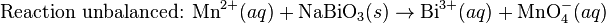$\mbox{Reaction unbalanced: }\mbox{Mn}^{2+}(aq) + \mbox{NaBiO}_3(s)\rightarrow\mbox{Bi}^{3+}(aq) + \mbox{MnO}_4^{-}(aq)\,$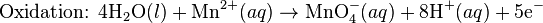$\mbox{Oxidation: }\mbox{4H}_2\mbox{O}(l)+\mbox{Mn}^{2+}(aq)\rightarrow\mbox{MnO}_4^{-}(aq) + \mbox{8H}^{+}(aq)+\mbox{5e}^{-}\,$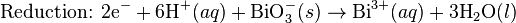$\mbox{Reduction: }\mbox{2e}^{-}+ \mbox{6H}^{+}(aq) + \mbox{BiO}_3^{-}(s)\rightarrow\mbox{Bi}^{3+}(aq) + \mbox{3H}_2\mbox{O}(l)\,$

Finally the reaction is balanced by multiplying the number of electrons from the reduction half reaction to oxidation half reaction and vice versa and adding both half reactions, thus solving the equation.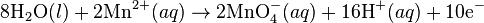$\mbox{8H}_2\mbox{O}(l)+\mbox{2Mn}^{2+}(aq)\rightarrow\mbox{2MnO}_4^{-}(aq) + \mbox{16H}^{+}(aq)+\mbox{10e}^{-}\,$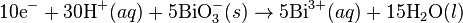$\mbox{10e}^{-}+ \mbox{30H}^{+}(aq) + \mbox{5BiO}_3^{-}(s)\rightarrow\mbox{5Bi}^{3+}(aq) + \mbox{15H}_2\mbox{O}(l)\,$

Reaction balanced: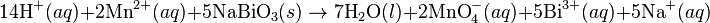$\mbox{14H}^{+}(aq) + \mbox{2Mn}^{2+}(aq)+ \mbox{5NaBiO}_3(s)\rightarrow\mbox{7H}_2\mbox{O}(l) + \mbox{2MnO}_4^{-}(aq)+\mbox{5Bi}^{3+}(aq)+\mbox{5Na}^{+}(aq)\,$

#### Basic medium

In basic medium OH- ions and water are added to half reactions to balance the overall reaction. For example on reaction between Potassium permanganate and Sodium sulfite.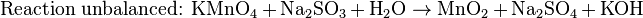$\mbox{Reaction unbalanced: }\mbox{KMnO}_{4}+\mbox{Na}_{2}\mbox{SO}_3+\mbox{H}_2\mbox{O}\rightarrow\mbox{MnO}_{2}+\mbox{Na}_{2}\mbox{SO}_{4}+\mbox{KOH}\,$$\mbox{Reduction: }\mbox{3e}^{-}+\mbox{2H}_{2}\mbox{O}+\mbox{MnO}_{4}^{-}\rightarrow\mbox{MnO}_{2}+\mbox{4OH}^{-}\,$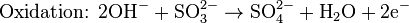$\mbox{Oxidation: }\mbox{2OH}^{-}+\mbox{SO}^{2-}_{3}\rightarrow\mbox{SO}^{2-}_{4}+\mbox{H}_{2}\mbox{O}+\mbox{2e}^{-}\,$

The same procedure as followed on acid medium by multiplying electrons to opposite half reactions solve the equation thus balancing the overall reaction.$\mbox{6e}^{-}+\mbox{4H}_{2}\mbox{O}+\mbox{2MnO}_{4}^{-}\rightarrow\mbox{2MnO}_{2}+\mbox{8OH}^{-}\,$$\mbox{6OH}^{-}+\mbox{3SO}^{2-}_{3}\rightarrow\mbox{3SO}^{2-}_{4}+\mbox{3H}_{2}\mbox{O}+\mbox{6e}^{-}\,$

Equation balanced:$\mbox{2KMnO}_{4}+\mbox{3Na}_{2}\mbox{SO}_3+\mbox{H}_2\mbox{O}\rightarrow\mbox{2MnO}_{2}+\mbox{3Na}_{2}\mbox{SO}_{4}+\mbox{2KOH}\,$

#### Neutral medium

The same procedure as used on acid medium is applied, for example on balancing using electron ion method to complete combustion of propane.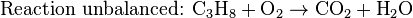$\mbox{Reaction unbalanced: }\mbox{C}_{3}\mbox{H}_{8}+\mbox{O}_{2}\rightarrow\mbox{CO}_{2}+\mbox{H}_{2}\mbox{O}\,$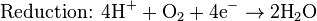$\mbox{Reduction: }\mbox{4H}^{+} + \mbox{O}_{2}+ \mbox{4e}^{-}\rightarrow\mbox{2H}_{2}\mbox{O}\,$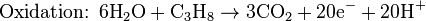$\mbox{Oxidation: }\mbox{6H}_{2}\mbox{O}+\mbox{C}_{3}\mbox{H}_{8}\rightarrow\mbox{3CO}_{2}+\mbox{20e}^{-}+\mbox{20H}^{+}\,$

As in acid and basic medium, electrons which were used to compensate oxidation changes are multiplied to opposite half reactions, thus solving the equation.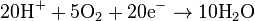$\mbox{20H}^{+}+\mbox{5O}_{2}+\mbox{20e}^{-}\rightarrow\mbox{10H}_{2}\mbox{O}\,$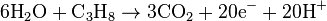$\mbox{6H}_{2}\mbox{O}+\mbox{C}_{3}\mbox{H}_{8}\rightarrow\mbox{3CO}_{2}+\mbox{20e}^{-}+\mbox{20H}^{+}\,$

Equation balanced:$\mbox{C}_{3}\mbox{H}_{8}+\mbox{5O}_{2}\rightarrow\mbox{3CO}_{2}+\mbox{4H}_{2}\mbox{O}\,$

## Electrochemical cells

An electrochemical cell is a device that produces an electric current from energy released by a spontaneous redox reaction. This kind of cell includes the Galvanic cell or Voltaic cell, named after Luigi Galvani and Alessandro Volta, both scientists who conducted several experiments on chemical reactions and electric current during the late eighteenth century.

Electrochemical cells have two conductive electrodes (the anode and the cathode). The anode is defined as the electrode where oxidation occurs and the cathode is the electrode where the reduction takes place. Electrodes can be made from any sufficiently conductive materials, such as metals, semiconductors, graphite, and even conductive polymers. In between these electrodes is the electrolyte, which contains ions that can freely move.

The Galvanic cell uses two different metal electrodes, each in an electrolyte where the positively charged ions are the oxidized form of the electrode metal. One electrode will undergo oxidation (the anode) and the other will undergo reduction (the cathode). The metal of the anode will oxidize, going from an oxidation state of 0 (in the solid form) to a positive oxidation state and become an ion. At the cathode, the metal ion in solution will accept one or more electrons from the cathode and the ion's oxidation state is reduced to 0. This forms a solid metal that electrodeposits on the cathode. The two electrodes must be electrically connected to each other, allowing for a flow of electrons that leave the metal of the anode and flow through this connection to the ions at the surface of the cathode. This flow of electrons is an electrical current that can be used to do work, such as turn a motor or power a light.

A Galvanic cell whose electrodes are zinc and copper submerged in zinc sulfate and copper sulfate, respectively, is known as a Daniell cell.

Half reactions for a Daniell cell are these: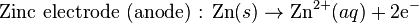$\mbox{Zinc electrode (anode) : }\mbox{Zn}(s)\rightarrow\mbox{Zn}^{2+}(aq)+\mbox{2e}^{-}\,$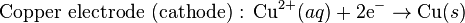$\mbox{Copper electrode (cathode) : }\mbox{Cu}^{2+}(aq)+\mbox{2e}^{-}\rightarrow\mbox{Cu}(s)\,$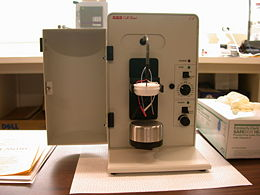A modern cell stand for electrochemical research. The electrodes attach to high-quality metallic wires, and the stand is attached to a potentiostat/galvanostat (not pictured). A shotglass-shaped container is aerated with a noble gas and sealed with the Teflon block.

In this example, the anode is zinc metal which oxidizes (loses electrons) to form zinc ions in solution, and copper ions accept electrons from the copper metal electrode and the ions deposit at the copper cathode as an electrodeposit. This cell forms a simple battery as it will spontaneously generate a flow of electrical current from the anode to the cathode through the external connection. This reaction can be driven in reverse by applying a voltage, resulting in the deposition of zinc metal at the anode and formation of copper ions at the cathode.

To provide a complete electric circuit, there must also be an ionic conduction path between the anode and cathode electrolytes in addition to the electron conduction path. The simplest ionic conduction path is to provide a liquid junction. To avoid mixing between the two electrolytes, the liquid junction can be provided through a porous plug that allows ion flow while reducing electrolyte mixing. To further minimize mixing of the electrolytes, a salt bridge can be used which consists of an electrolyte saturated gel in an inverted U-tube. As the negatively charged electrons flow in one direction around this circuit, the positively charged metal ions flow in the opposite direction in the electrolyte.

A voltmeter is capable of measuring the change of electrical potential between the anode and the cathode.

Electrochemical cell voltage is also referred to as electromotive force or emf.

A cell diagram can be used to trace the path of the electrons in the electrochemical cell. For example, here is a cell diagram of a Daniell cell: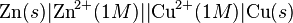$\mbox{Zn}(s)|\mbox{Zn}^{2+}(1M)||\mbox{Cu}^{2+}(1M)|\mbox{Cu}(s)\,$

First, the reduced form of the metal to be oxidized at the anode (Zn) is written. This is separated from its oxidized form by a vertical line, which represents the limit between the phases (oxidation changes). The double vertical lines represent the saline bridge on the cell. Finally, the oxidized form of the metal to be reduced at the cathode, is written, separated from its reduced form by the vertical line. The electrolyte concentration is given as it is an important variable in determining the cell potential.

## Standard electrode potential

To allow prediction of the cell potential, tabulations of standard electrode potential are available. Such tabulations are referenced to the standard hydrogen electrode (SHE). The standard hydrogen electrode undergoes the reaction$\mbox{2H}^{+}(aq) + \mbox{2e}^{-} \rightarrow \mbox{H}_{2}\,$

which is shown as reduction but, in fact, the SHE can act as either the anode or the cathode, depending on the relative oxidation/reduction potential of the other electrode/electrolyte combination. The term standard in SHE requires a supply of hydrogen gas bubbled through the electrolyte at a pressure of 1 atm and an acidic electrolyte with H+ activity equal to 1 (usually assumed to be [H+] = 1 mol/liter).

The SHE electrode can be connected to any other electrode by a salt bridge to form a cell. If the second electrode is also at standard conditions, then the measured cell potential is called the standard electrode potential for the electrode. The standard electrode potential for the SHE is zero, by definition. The polarity of the standard electrode potential provides information about the relative reduction potential of the electrode compared to the SHE. If the electrode has a positive potential with respect to the SHE, then that means it is a strongly reducing electrode which forces the SHE to be the anode (an example is Cu in aqueous CuSO4 with a standard electrode potential of 0.337 V). Conversely, if the measured potential is negative, the electrode is more oxidizing than the SHE (such as Zn in ZnSO4 where the standard electrode potential is -0.763 V).

Standard electrode potentials are usually tabulated as reduction potentials. However, the reactions are reversible and the role of a particular electrode in a cell depends on the relative oxidation/reduction potential of both electrodes. The oxidation potential for a particular electrode is just the negative of the reduction potential. A standard cell potential can be determined by looking up the standard electrode potentials for both electrodes (sometimes called half cell potentials). The one that is smaller will be the anode and will undergo oxidation. The cell potential is then calculated as the sum of the reduction potential for the cathode and the oxidation potential for the anode.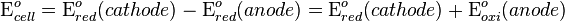$\mbox{E}^{o}_{cell}=\mbox{E}^{o}_{red}(cathode)-\mbox{E}^{o}_{red}(anode) = \mbox{E}^{o}_{red}(cathode)+\mbox{E}^{o}_{oxi}(anode)$

For example, the standard electrode potential for a copper electrode is:$\mbox{Cell diagram}\,$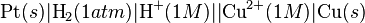$\mbox{Pt}(s)|\mbox{H}_{2}(1 atm)|\mbox{H}^{+}(1 M)||\mbox{Cu}^{2+}(1 M)|\mbox{Cu}(s)\,$$\mbox{E}^{o}_{cell}=\mbox{E}^{o}_{red}(cathode)-\mbox{E}^{o}_{red}(anode)$

At standard temperature, pressure and concentration conditions, the cell's emf (measured by a multimeter) is 0.34 V. by definition, the electrode potential for the SHE is zero. Thus, the Cu is the cathode and the SHE is the anode giving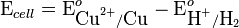$\mbox{E}_{cell}=\mbox{E}^{o}_{\mbox{Cu}^{2+}/\mbox{Cu}}-\mbox{E}^{o}_{\mbox{H}^{+}/\mbox{H}_{2}}$

Or,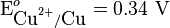$\mbox{E}^{o}_{\mbox{Cu}^{2+}/\mbox{Cu}} = \mbox{0.34 V}$

Changes in the stoichiometric coefficients of a balanced cell equation will not change$\mbox{E}^{0}_{red}\,$ value because the standard electrode potential is an intensive property.

## Spontaneity of Redox reaction

During operation of electrochemical cells, chemical energy is transformed into electrical energy and is expressed mathematically as the product of the cell's emf and the electrical charge transferred through the external circuit.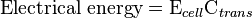$\mbox{Electrical energy}=\mbox{E}_{cell} \mbox{C}_{trans}\,$

where$\mbox{E}_{cell}\,$ is the cell potential measured in volts (V) and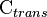$\mbox{C}_{trans}\,$ is the cell current integrated over time and measured in coulumbs (C).$\mbox{C}_{trans}\,$ can also be determined by multiplying the total number of electrons transferred (measured in moles) times Faraday's constant, F = 96,485 C/mole.

The emf of the cell at zero current is the maximum possible emf. It is used to calculate the maximum possible electrical energy that could be obtained from a chemical reaction. This energy is referred to as electrical work and is expressed by the following equation: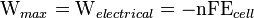$\mbox{W}_{max}=\mbox{W}_{electrical} = -\mbox{nFE}_{cell}\,$

where work is defined as positive into the system.

Since the free energy is the maximum amount of work that can be extracted from a system, one can write: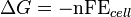$\Delta G=-\mbox{nFE}_{cell}\,$

A positive cell potential gives a negative change in Gibbs free energy. This is consistent with the cell production of an electric current flowing from the cathode to the anode through the external circuit. If the current is driven in the opposite direction by imposing an external potential, then work is done on the cell to drive electrolysis.

A spontaneous electrochemical reaction (change in Gibbs free energy less than zero) can be used to generate an electric current, in electrochemical cells. This is the basis of all batteries and fuel cells. For example, gaseous oxygen (O2) and hydrogen (H2) can be combined in a fuel cell to form water and energy, typically a combination of heat and electrical energy.

Conversely, non-spontaneous electrochemical reactions can be driven forward by the application of a current at sufficient voltage. The electrolysis of water into gaseous oxygen and hydrogen is a typical example.

The relation between the equilibrium constant, K, and the Gibbs free energy for an electrochemical cell is expressed as follows: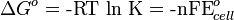$\Delta G^{o}=\mbox{-RT ln K}= \mbox{-nFE}^{o}_{cell}\,$

Rearranging to express the relation between standard potential and equilibrium constant yields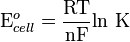$\mbox{E}^{o}_{cell}={\mbox{RT} \over \mbox{nF}} \mbox{ln K}\,$

Previous equation can use Briggsian logarithm as shown below:$\mbox{E}^{o}_{cell}={0.0592 \mbox{V} \over \mbox{n}} \mbox{log K}\,$

## Cell emf dependency on changes in concentration

### Nernst Equation

The standard potential of an electrochemical cell requires standard conditions for all of the reactants. When reactant concentrations differ from standard conditions, the cell potential will deviate from the standard potential. In the twentieth century German chemist Walther Hermann Nernst proposed a mathematical model to determine the effect of reactant concentration on electrochemical cell potential.

In the late nineteenth century Josiah Willard Gibbs had formulated a theory to predict whether a chemical reaction is spontaneous based on the free energy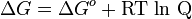$\Delta G=\Delta G^{o}+\mbox{RT ln Q}\,$ ,

Where:

ΔG = change in Gibbs free energy, T = absolute temperature, R = gas constant, ln = natural logarithm, Q = reaction quotient.

Gibbs' key contribution was to formalize the understanding of the effect of reactant concentration on spontaneity.

Based on Gibbs' work, Nernst extended the theory to include the contribution from electric potential on charged species. As shown in the previous section, the change in Gibbs free energy for an electrochemical cell can be related to the cell potential. Thus, Gibbs' theory becomes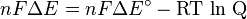$nF\Delta E = nF\Delta E^\circ - \mbox{RT ln Q} \,$

Where:

n = number of electrons/mole product, F = Faraday constant (coulombs/mole), and ΔE = cell potential.

Finally, Nernst divided through by the amount of charge transferred to arrive at a new equation which now bears his name: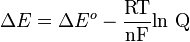$\Delta E=\Delta E^{o}- {\mbox{RT} \over \mbox{nF}} \mbox{ln Q}\,$

Assuming standard conditions (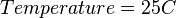$Temperature = 25 C\,$) and R =$8.3145 {J \over K mol}$ the equation above can be expressed on Base—10 logarithm as shown below:$\Delta E=\Delta E^{o}- {\mbox{0.0592 V} \over \mbox{n}} \mbox{log Q}\,$

### Concentration cells

A concentration cell is an electrochemical cell where the two electrodes are the same material, the electrolytes on the two half-cells involve the same ions, but the electrolyte concentration differs between the two half-cells.

For example an electrochemical cell, where two copper electrodes are submerged in two copper(II) sulfate solutions, whose concentrations are 0.05 M and 2.0 M, connected through a salt bridge. This type of cell will generate a potential that can be predicted by the Nernst equation. Both electrodes undergo the same chemistry (although the reaction proceeds in reverse at the cathode)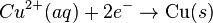$Cu^{2+}(aq)+2e^{-}\rightarrow \mbox{Cu}(s)$

Le Chatelier's principle indicates that the reaction is more favorable to reduction as the concentration of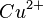$Cu^{2+}\,$ ions increases. Reduction will take place in the cell's compartment where concentration is higher and oxidation will occur on the more dilute side.

The following cell diagram describes the cell mentioned above: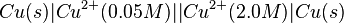$Cu(s)|Cu^{2+}(0.05 M)||Cu^{2+}(2.0 M)|Cu(s)\,$

Where the half cell reactions for oxidation and reduction are: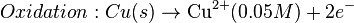$Oxidation: Cu(s)\rightarrow \mbox{Cu}^{2+} (0.05 M) + 2e^{-}\,$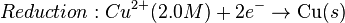$Reduction: Cu^{2+} (2.0 M) +2e^{-} \rightarrow \mbox{Cu} (s)\,$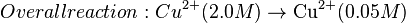$Overall reaction: Cu^{2+} (2.0 M) \rightarrow \mbox{Cu}^{2+} (0.05 M)\,$

Where the cell's emf is calculated through Nernst equation as follows:$E = E^{o}- {0.0257 V \over 2} ln {[Cu^{2+}]_{diluted}\over [Cu^{2+}]_{concentrated}}\,$$E^{o}\,$'s value of this kind of cell is zero, as electrodes and ions are the same in both half-cells. After replacing values from the case mentioned, it is possible to calculate cell's potential:$E = 0- {0.0257 V \over 2} ln {0.05\over 2.0}= 0.0474{ } V\,$

However, this value is only approximate, as reaction quotient is defined in terms of ion activities which can be approximated with the concentrations as calculated here.

The Nernst equation plays an important role in understanding electrical effects in cells and organelles. Such effects include nerve synapses and cardiac beat as well as the resting potential of a somatic cell.

## Battery

Main article: Battery (electricity)

A battery is an electrochemical cell (sometimes several in series) used for chemical energy storage. Batteries are optimized to produce a constant electric current for as long as possible. Although the cells discussed previously are useful for theoretical purposes and some laboratory experiments, the large internal resistance of the salt bridge make them inappropriate battery technologies. Various alternative battery technologies have been commercialized as discussed next.

### Dry cell

Dry cells do not have a fluid electrolyte. Instead, they use a moist electrolyte paste. Leclanché's cell is a good example of this, where the anode is a zinc container surrounded by a thin layer of manganese dioxide and a moist electrolyte paste of ammonium chloride and zinc chloride mixed with starch. The cell's cathode is represented by a carbon bar inserted on the cell's electrolyte, usually placed in the middle.

Leclanché's simplified half reactions are shown below: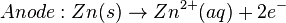$Anode: Zn(s) \rightarrow Zn^{2+} (aq) + 2e^{-}\,$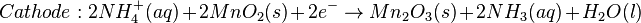$Cathode: 2NH^{+}_{4}(aq)+ 2MnO_{2}(s) + 2e^{-}\rightarrow Mn_{2}O_{3}(s) + 2NH_{3} (aq) + H_{2}O (l)\,$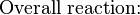$\mbox{Overall reaction:}\,$$Zn(s) + 2NH^{+}_{4}(aq)+ 2MnO_{2}(s) \rightarrow Zn^{2+}(aq) + Mn_{2}O_{3}(s) + 2NH_{3} (aq) + H_{2}O (l)\,$

The voltage obtained from the zinc-carbon battery is around 1.5 V.

### Mercury battery

The mercury battery has many applications in medicine and electronics. The battery consists of a steel—made container in the shape of a cylinder acting as the cathode, where an amalgamated anode of mercury and zinc is surrounded by a stronger alkaline electrolyte and a paste of zinc oxide and mercury(II) oxide.

Mercury battery half reactions are shown below:$Anode: Zn(Hg) + 2OH^{-} (aq) \rightarrow ZnO(s) + H_{2}O (l) + 2e^{-}\,$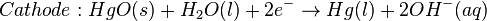$Cathode: HgO(s) + H_{2}O(l) + 2e^{-}\rightarrow Hg(l) + 2OH^{-} (aq)\,$$\mbox{Overall reaction:}\,$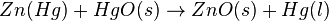$Zn(Hg) + HgO(s) \rightarrow ZnO(s) + Hg(l)\,$

There are no changes in the electrolyte's composition when the cell works. Such batteries provide 1.35 V of direct current.

The lead-acid battery used in automobiles, consists of a series of six identical cells assembled in series. Each cell has a lead anode and a cathode made from lead dioxide packed in a metal plaque. Cathode and anode are submerged in a solution of sulfuric acid acting as the electrolyte.

Lead-acid battery half cell reactions are shown below: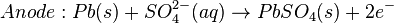$Anode: Pb(s) + SO^{2-}_{4}(aq) \rightarrow PbSO_{4}(s) + 2e^{-}\,$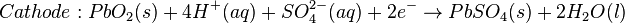$Cathode: PbO_{2}(s) + 4H^{+}(aq) + SO^{2-}_{4}(aq) + 2e^{-} \rightarrow PbSO_{4}(s) + 2H_{2}O(l)\,$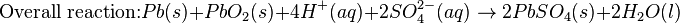$\mbox{Overall reaction:} Pb(s) + PbO_{2}(s) + 4H^{+}(aq)+2SO^{2-}_{4}(aq) \rightarrow 2PbSO_{4}(s) + 2H_{2}O(l)$

At standard conditions, each cell may produce a potential of 2 V, hence overall voltage produced is 12 V. Differing from mercury and zinc-carbon batteries, lead-acid batteries are rechargeable. If an external voltage is supplied to the battery it will produce an electrolysis of the products in the overall reaction (discharge), thus recovering initial components which made the battery work.

### Lithium rechargeable battery

Instead of an aqueous electrolyte or a moist electrolyte paste, a solid state battery operates using a solid electrolyte. Lithium polymer batteries are an example of this; a graphite bar acts as the anode, a bar of lithium cobaltate acts as the cathode, and a polymer, swollen with a lithium salt, allows the passage of ions and serves as the electrolyte. In this cell, the carbon in the anode can reversibly form a lithium-carbon alloy. Upon discharging, lithium ions spontaneously leave the lithium cobaltate cathode and travel through the polymer and into the carbon anode forming the alloy. This flow of positive lithium ions is the electrical current that the battery provides. By charging the cell, the lithium dealloys and travels back into the cathode. The advantage of this kind of battery is that Lithium possesses the highest negative value of standard reduction potential. It is also a light metal and therefore less mass is required to generate 1 mole of electrons. Lithium ion battery technologies are widely used in portable electronic devices because they have high energy storage density and are rechargeable. These technologies show promise for future automotive applications, with new materials such as iron phosphates and lithium vanadates.

### Flow battery/ Redox flow battery

Most batteries have all of the electrolyte and electrodes within a single housing. A flow battery is unusual in that the majority of the electrolyte, including dissolved reactive species, is stored in separate tanks. The electrolytes are pumped through a reactor, which houses the electrodes, when the battery is charged or discharged.

These types of batteries are typically used for large-scale energy storage (kWh - multi MWh). Of the several different types that have been developed, some are of current commercial interest, including the vanadium redox battery and zinc bromine battery.

### Fuel cells

Main article: Fuel cell

Fossil fuels are used in power plants to supply electrical needs, however their conversion into electricity is an inefficient process. The most efficient electrical power plant may only convert about 40 percent of the original chemical energy into electricity when burned or processed.

To enhance electrical production, scientists have developed fuel cells where combustion is replaced by electrochemical methods, similar to a battery but requiring continuous replenishment of the reactants consumed.

The most popular is the oxygen-hydrogen fuel cell, where two inert electrodes (porous electrodes of nickel and nickel oxide) are placed in an electrolytic solution such as hot caustic potash, in both compartments (anode and cathode) gaseous hydrogen and oxygen are bubbled into solution.

Oxygen-hydrogen fuel cell reactions are shown below: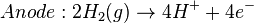$Anode: 2H_{2}(g)\rightarrow 4H^{+}+4e^{-}\,$$Cathode: O_{2}(g)+ 4e^{-} + 4 H^{+}\rightarrow 2H_{2}O(l)\,$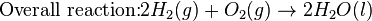$\mbox{Overall reaction:} 2H_{2}(g) + O_{2}(g)\rightarrow 2H_{2}O(l)\,$

The overall reaction is identical to hydrogen combustion. Oxidation and reduction take place in the anode and cathode separately. This is similar to the electrode used in the cell for measuring standard reduction potential which has a double function acting as electrical conductors providing a surface required to decomposition of the molecules into atoms before electron transferring, thus named electrocatalysts. Platinum, nickel, and rhodium are good electrocatalysts.

## Corrosion

Main article: Corrosion

Corrosion is the term applied to metal rust caused by an electrochemical process. Most people are likely familiar with the corrosion of iron, in the form of reddish rust. Other examples include the black tarnish on silver, and red or green corrosion that may appear on copper and its alloys, such as brass. The cost of replacing metals lost to corrosion is in the multi-billions of dollars per year.

### Iron corrosion

For iron rust to occur the metal has to be in contact with oxygen and water, although chemical reactions for this process are relatively complex and not all of them are completely understood, it is believed the causes are the following:

1. Electron transferring (Reduction-Oxidation)
1. One area on the surface of the metal acts as the anode, which is where the oxidation (corrosion) occurs. At the anode, the metal gives up electrons.
1.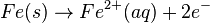$Fe(s)\rightarrow Fe^{2+}(aq) + 2e^{-}\,$
2. Electrons are transferred from iron reducing oxygen in the atmosphere into water on the cathode, which is placed in another region of the metal.
1.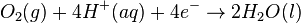$O_{2}(g) + 4H^{+}(aq) + 4e^{-} \rightarrow 2H_{2}O(l)\,$
3. Global reaction for the process: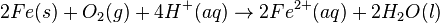$2Fe(s) + O_{2}(g) + 4H^{+}(aq) \rightarrow 2Fe^{2+}(aq) + 2H_{2}O(l)\,$
4. Standard emf for iron rusting:
1.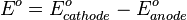$E^{o}=E^{o}_{cathode}-E^{o}_{anode}\,$$E^{o}=1.23V-(-0.44V)=1.67V\,$

Iron corrosion takes place on acid medium; H+ ions come from reaction between carbon dioxide in the atmosphere and water, forming carbonic acid. Fe2+ ions oxides, following this equation:$4Fe^{2+}(aq) + O_{2}(g) + (4+2x)H_{2}O(l) \rightarrow 2Fe_{2}O_{3}.xH_{2}O + 8H^{+}(aq)$

Iron(III) oxide hydrated is known as rust. The concentration of water associated with iron oxide varies, thus chemical representation is presented as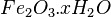$Fe_{2}O_{3}.xH_{2}O\,$. The electric circuit works as passage of electrons and ions occurs, thus if an electrolyte is present it will facilitate oxidation, this explains why rusting is quicker on salt water.

### Corrosion of common metals

Coinage metals, such as copper and silver, slowly corrode through use. A patina of green-blue copper carbonate forms on the surface of copper with exposure to the water and carbon dioxide in the air. Silver coins or cutlery that are exposed to high sulfur foods such as eggs or the low levels of sulfur species in the air develop a layer of black Silver sulfide.

Gold and platinum are extremely difficult to oxidize under normal circumstances, and require exposure to a powerful chemical oxidizing agent such as aqua regia.

Some common metals oxidize extremely rapidly in air. Titanium and aluminium oxidize instantaneouly in contact with the oxygen in the air. These metals form an extremely thin layer of oxidized metal on the surface. This thin layer of oxide protects the underlying layers of the metal from the air preventing the entire metal from oxidizing. These metals are used in applications where corrosion resistance is important. Iron, in contrast, has an oxide that forms in air and water, called rust, that does not stop the further oxidation of the iron. Thus iron left exposed to air and water will continue to rust until all of the iron is oxidized.

### Prevention of corrosion

Attempts to save a metal from becoming anodic are of two general types. Anodic regions dissolve and destroy the structural integrity of the metal.

While it is almost impossible to prevent anode/cathode formation, if a non-conducting material covers the metal, contact with the electrolyte is not possible and corrosion will not occur.

#### Coating

Metals are coated on its surface with paint or some other non-conducting coating. This prevents the electrolyte from reaching the metal surface if the coating is complete. Scratches exposing the metal will corrode with the region under the paint, adjacent to the scratch, to be anodic.

Other prevention is called passivation where a metal is coated with another metal such as a tin can. Tin is a metal that rapidly corrodes to form a mono-molecular oxide coating that prevents further corrosion of the tin. The tin prevents the electrolyte from reaching the base metal, usually steel (iron). However, if the tin coating is scratched the iron becomes anodic and corrodes rapidly.

#### Sacrificial anodes

A method commonly used to protect a structural metal is to attach a metal which is more anodic than the metal to be protected. This forces the structural metal to be cathodic, thus spared corrosion. It is called "sacrificial" because the anode dissolves and has to be replaced periodically.

Zinc bars are attached at various locations on steel ship hulls to render the ship hull cathodic. The zinc bars are replaced periodically. Other metals, such as magnesium, would work very well but zinc is the least expensive useful metal.

To protect pipelines, an ingot of buried or exposed magnesium (or zinc) is buried beside the pipeline and is connected electrically to the pipe above ground. The pipeline is forced to be a cathode and is protected from being oxidized and rusting. The magnesium anode is sacrificed. At intervals new ingots are buried to replace those lost.

## Electrolysis

Main article: Electrolysis

The spontaneous redox reactions of a conventional battery produce electricity through the different chemical potentials of the cathode and anode in the electrolyte. However, electrolysis requires an external source of electrical energy to induce a chemical reaction, and this process takes place in a compartment called an electrolytic cell.

### Electrolysis of molten sodium chloride

When molten, the salt sodium chloride can be electrolyzed to yield metallic sodium and gaseous chlorine. Industrially, this process is carried out in a special cell called a Downs cell. The cell is connected to an electrical power supply, allowing electrons to migrate from the power supply to the electrolytic cell.

Reactions that take place in the cell are the following:$\mbox{Anode (oxidation): }2Cl^{-} \rightarrow Cl_{2}(g) + 2e^{-}\,$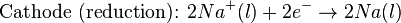$\mbox{Cathode (reduction): }2Na^{+}(l) + 2e^{-} \rightarrow 2Na(l)\,$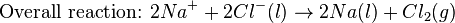$\mbox{Overall reaction: }2Na^{+} + 2Cl^{-}(l) \rightarrow 2Na(l) + Cl_{2}(g)\,$

This process can yield large amounts of metallic sodium and gaseous chlorine, and is widely used on mineral dressing and metallurgy industries.

The emf for this process is approximately -4 V indicating a (very) non-spontaneous process. In order for this reaction to occur the power supply should provide at least a potential of 4 V. However, larger voltages must be used for this reaction to occur at a high rate.

### Electrolysis of water

Water can be converted to its component elemental gasses, H2 and O2 through the application of an external voltage. Water doesn't decompose into hydrogen and oxygen spontaneously as the Gibbs free energy for the process at standard conditions is about 474.4 kJ. The decomposition of water into hydrogen and oxygen can be performed in an electrolytic cell. In it, a pair of inert electrodes usually made of platinum immersed in water act as anode and cathode in the electrolytic process. The electrolysis starts with the application of an external voltage between the electrodes. This process will not occur except at extremely high voltages without an electrolyte such as sodium chloride or sulfuric acid (most used 0.1 M).

Bubbles from the gases will be seen near both electrodes. The following half reactions describe the process mentioned above:$\mbox{Anode (oxidation): }2H_{2}O(l) \rightarrow O_{2}(g) + 4H^{+}(aq) + 4e^{-}\,$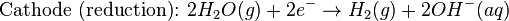$\mbox{Cathode (reduction): }2H_{2}O(g) + 2e^{-} \rightarrow H_{2}(g) + 2OH^{-}(aq)\,$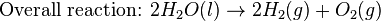$\mbox{Overall reaction: }2H_{2}O(l) \rightarrow 2H_{2}(g) + O_{2}(g)\,$

Although strong acids may be used in the apparatus, the reaction will not net consume the acid. While this reaction will work at any conductive electrode at a sufficiently large potential, platinum catalyzes both hydrogen and oxygen formation, allowing for relatively mild voltages (~2V depending on the pH).

### Electrolysis of aqueous solutions

Electrolysis in an aqueous is a similar process as mentioned in electrolysis of water. However, it is considered to be a complex process because the contents in solution have to be analyzed in half reactions, whether reduced or oxidized.

#### Electrolysis of a solution of sodium chloride

The presence of water in a solution of sodium chloride must be examined with respect to its reduction and oxidation in both electrodes. Usually, water is electrolyzed, as mentioned above (in the electrolysis of water), yielding gaseous oxygen in the anode and gaseous hydrogen in the cathode. On the other hand, sodium chloride in water dissociates into Na+ and Cl- ions. The sodium ions are attracted to the cathode, where they are reduced to sodium metal. The chloride ions are attracted to the anode, where they are oxidized to chlorine gas.

The following half reactions describes the process mentioned: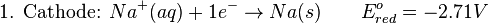$\mbox{1. Cathode: }Na^{+}(aq)+ 1e^{-} \rightarrow Na(s) \qquad E^{o}_{red}=-2.71 V\,$$\mbox{2. Anode: }2Cl^{-}(aq) \rightarrow Cl_{2}(g) + 2e^{-} \qquad E^{o}_{red}= +1.36 V\,$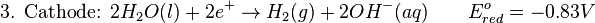$\mbox{3. Cathode: }2H_{2}O(l) + 2e^{+} \rightarrow H_{2}(g) + 2OH^{-}(aq)\qquad E^{o}_{red}=-0.83 V\,$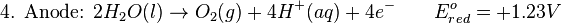$\mbox{4. Anode: } 2H_{2}O(l) \rightarrow O_{2}(g) + 4H^{+}(aq) + 4e^{-}\qquad E^{o}_{red}=+1.23V\,$

Reaction 1 is discarded, as it has the most negative value on standard reduction potential thus making it less thermodynamically favorable in the process.

When comparing the reduction potentials in reactions 2 & 4, the reduction of chloride ion is favored. Thus, if the Cl- ion is favored for reduction, then the water reaction is favored for oxidation producing gaseous oxygen, however experiments shown gaseous chlorine is produced and not oxygen.

Although the initial analysis is correct, there is another effect that can happen, known as the overvoltage effect. Additional voltage is sometimes required, beyond the voltage predicted by the$E^{o}_{cell}\,$. This may be due to kinetic rather than thermodynamic considerations. In fact, it has been proven that the activation energy for the chloride ion is very low, hence favorable in kinetic terms. In other words, although the voltage applied is thermodynamically sufficient to drive electrolysis, the rate is so slow that to make the process proceed in a reasonable time frame, the voltage of the external source has to be increased (hence, overvoltage).

Finally, reaction 3 is favorable because it describes the proliferation of OH- ions thus letting a probable reduction of H+ ions less favorable an option.

The overall reaction for the process according to the analysis would be the following: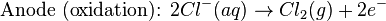$\mbox{Anode (oxidation): } 2Cl^{-}(aq)\rightarrow Cl_{2}(g) + 2e^{-}\,$$\mbox{Cathode (reduction): } 2H_{2}O(l) + 2e{-}\rightarrow H_{2}(g) + 2OH^{-}(aq)\,$$\mbox{Overall reaction: } 2H_{2}O + 2Cl^{-}(aq) \rightarrow H_{2}(g) + Cl_{2}(g) + 2OH^{-}(aq)\,$

As the overall reaction indicates, the concentration of chloride ions is reduced in comparison to OH- ions (whose concentration increases). The reaction also shows the production of gaseous hydrogen, chlorine and aqueous sodium hydroxide.

### Quantitative electrolysis & Faraday's Laws

Quantitative aspects of electrolysis were originally developed by Michael Faraday in 1834. Faraday is also credited with having coined the terms electrolyte and electrolysis, among many others while he studied quantitative analysis of electrochemical reactions. Also he was an advocate of the law of conservation of energy.

#### First law

Faraday concluded after several experiments on electrical current in non-spontaneous process, the mass of the products yielded on the electrodes was proportional to the value of current supplied to the cell, the length of time the current existed, and the molar mass of the substance analyzed.

In other words, the amount of a substance deposited on each electrode of an electrolytic cell is directly proportional to the quantity of electricity passed through the cell.

Below a simplified equation of Faraday's first law: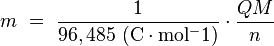$m \ = \ { 1 \over 96,485 \ \mathrm{(C \cdot mol^-1)} } \cdot { Q M \over n }$

Where,

m is the mass of the substance produced at the electrode (in grams),
Q is the total electric charge that passed through the solution (in coulombs),
n is the valence number of the substance as an ion in solution (electrons per ion),
M is the molar mass of the substance (in grams per mole).

#### Second law

Main article: Electroplating

Faraday devised the laws of chemical electrodeposition of metals from solutions in 1857. He formulated the second law of electrolysis stating "the amounts of bodies which are equivalent to each other in their ordinary chemical action have equal quantities of electricity naturally associated with them." In other terms, the quantities of different elements deposited by a given amount of electricity are in the ratio of their chemical equivalent weights.

An important aspect of the second law of electrolysis is electroplating which together with the first law of electrolysis, has a significant number of applications in the industry, as when used to protect metals to avoid corrosion.

## Applications

There are various extremely important electrochemical processes in both nature and industry. They include the coating of objects with metals or metal oxides through electrodeposition, and the detection of alcohol in drunken drivers through the redox reaction of ethanol. The generation of chemical energy through photosynthesis is inherently an electrochemical process, as is the production of metals like aluminum and titanium from their ores. Certain diabetes blood sugar meters measure the amount of glucose in the blood through its redox potential.

The nervous impulses in neurons are based on electric current generated by the movement of sodium and potassium ions into and out of cells. Some animals, such as eels, can generate a powerful voltage from certain cells that can disable much larger animals.